# Exam Questions for Chapters 5 and 6¶

The following questions test what you have learned in chapters 5 and 6. Click the “Start” button when you are ready to begin the exam. Click the “Pause” button to pause the exam (you will not be able to see the questions when the exam is paused). It will show how much time you have used, but you have unlimited time. Click on the “Finish Exam” button at the end when you are done. The number correct, number wrong, and number skipped will be displayed at the bottom of the page. Feedback for each answer will also be shown as well as your answer.

You will not be able to change your answers after you hit the “Finish Exam” button.

6-10-1: What value is printed when the following code is executed?

```name = "John Smith"
def myFunction(parameter):
value = "First"
value = parameter
print (value)

myFunction("Second")
```
• value
• When you print a variable it will print the value of the variable.
• Second
• When you call myFunction("Second") the value of parameter is set to "Second". This code prints the value of the variable called "value" which is set to the value of parameter.
• parameter
• When value = parameter is executed the value of parameter is copied into the space called value.
• First
• While value was first set to "First" it was changed to a copy of the value of parameter.

6-10-2: Which picture would the following code produce?

```from turtle import *
screen = Screen()
alice = Turtle()
alice.forward(50)
alice.left(90)
alice.forward(50)
alice.left(90)
alice.pencolor("red")
alice.forward(50)
alice.left(90)
alice.forward(50)
```
•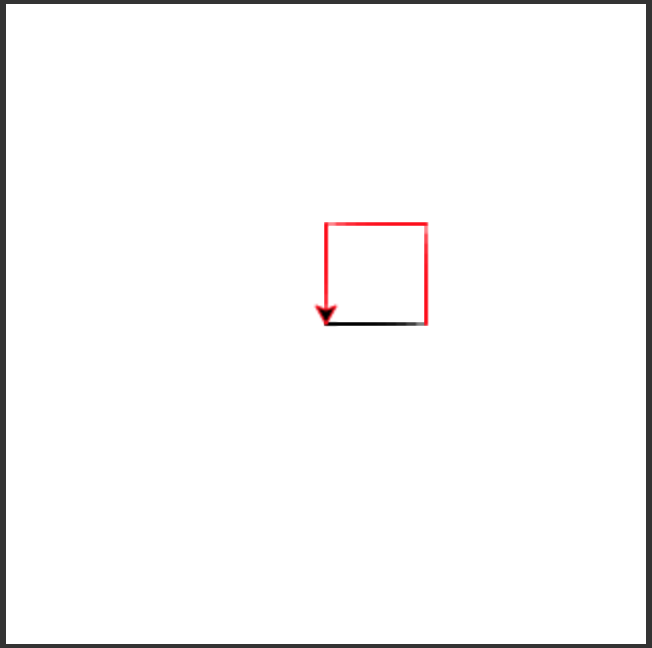• This would be true if the alice.pencolor("red") was after the first forward.
•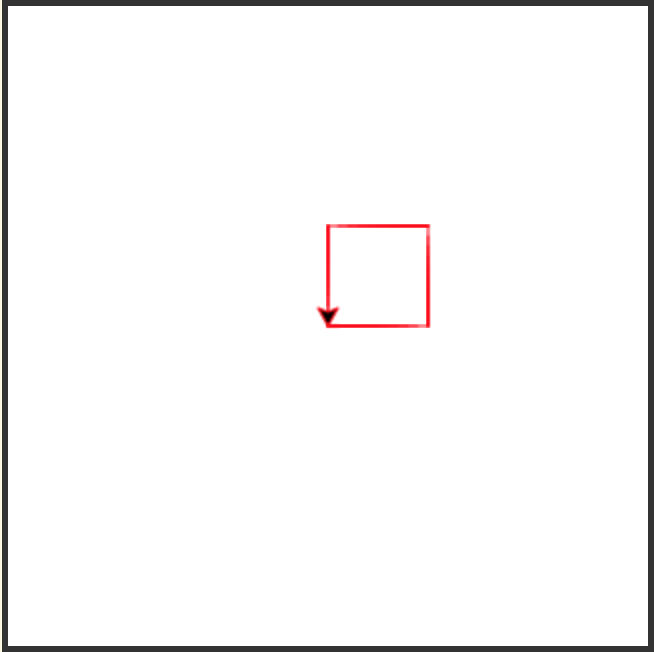• This would be true if the alice.pencolor("red") was before the first forward.
•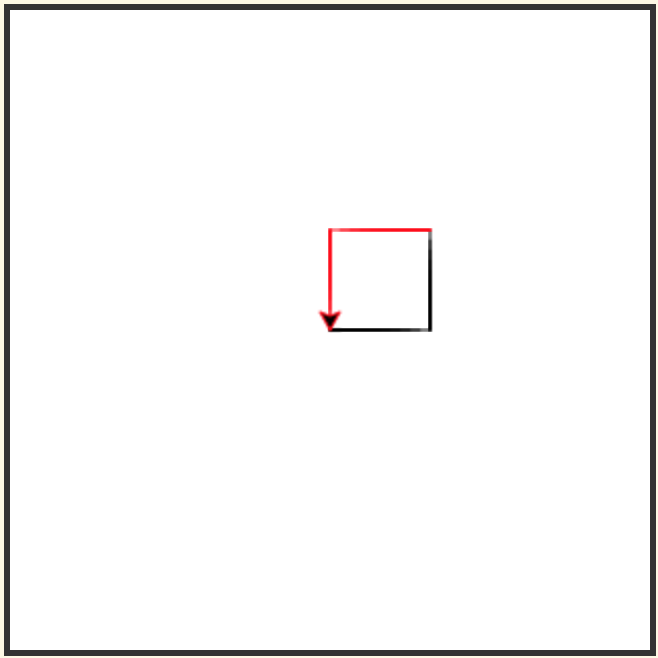• Since the alice.pencolor("red") is after the second forward the first two lines will be black and the last two will be red.
•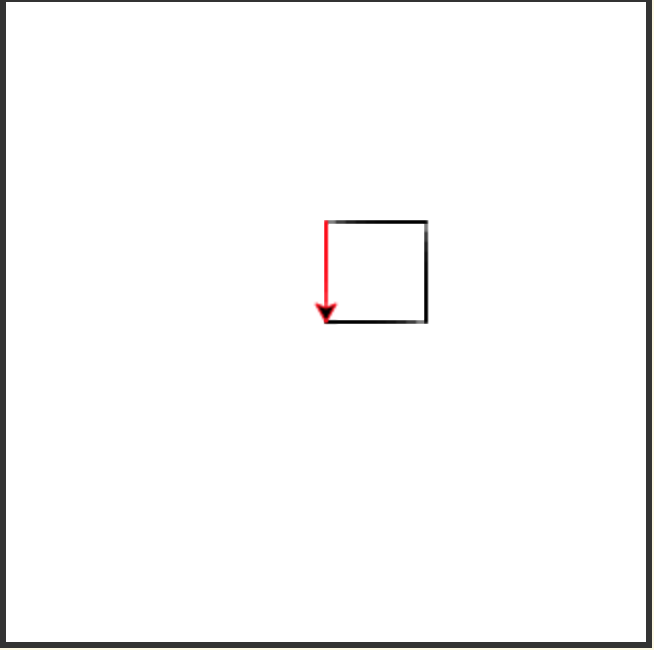• This would be true if the alice.pencolor("red") was after the third forward.

6-10-3: Given the following lines of code, which will move the turtle bob 50 units forward?

```from turtle import *
space = Screen()
bob = Turtle()
```
• bob.move(50)
• Turtles don't understand move.
• bob.left(50)
• The left procedure turns the turtle left by the specified amount.
• bob.forward(50)
• Turtles have a forward procedure which moves the turtle in the direction it is facing by the specified amount.
• bob.right(50)
• The right procedure turns the turtle right by the specified amount.

6-10-4: A named sequence of statements that returns a result is known as which of the following?

• definition
• You use the def keyword to define a procedure or function.
• procedure
• A procedure doesn't return anything.
• turtle
• Turtles have procedures and functions.
• function
• A function returns a result.

6-10-5: Which picture would the following code produce?

```from turtle import *
space = Screen()
sue = Turtle()
sue.left(90)
sue.forward(75)
sue.right(90)
sue.forward(150)
```
•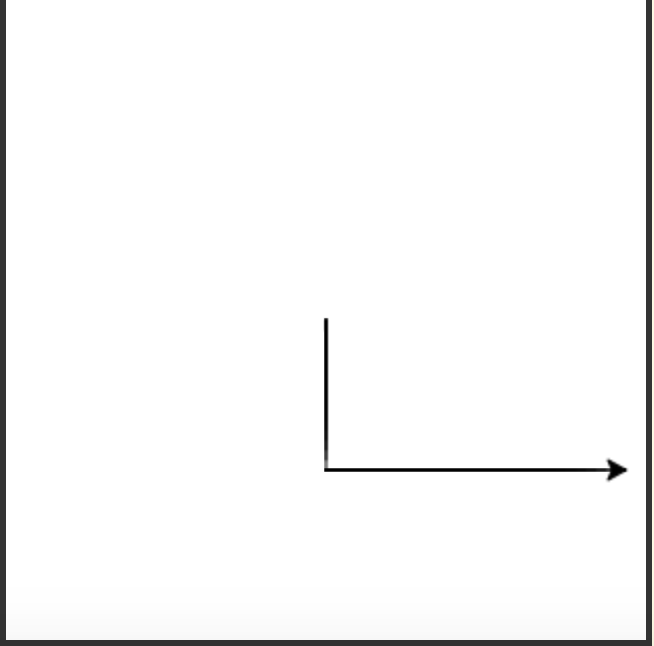• This would be true if it was right first and then left.
•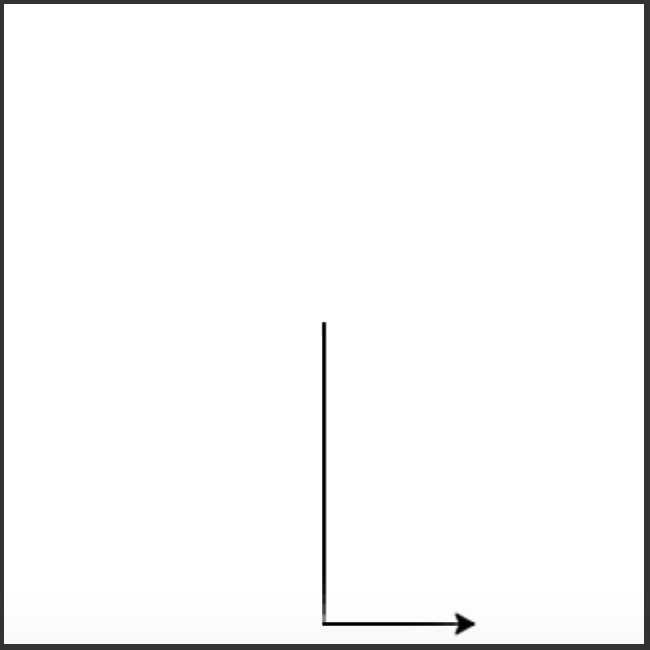• This would be true if it was right first and then left and if the first forward was 150 and the last was 75.
•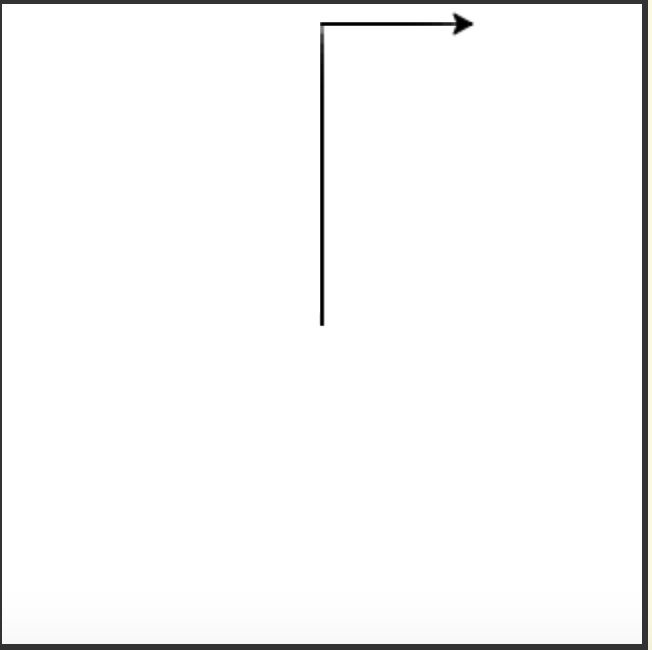• This would be true if it was the shorter line to the north and the longer to the east.
•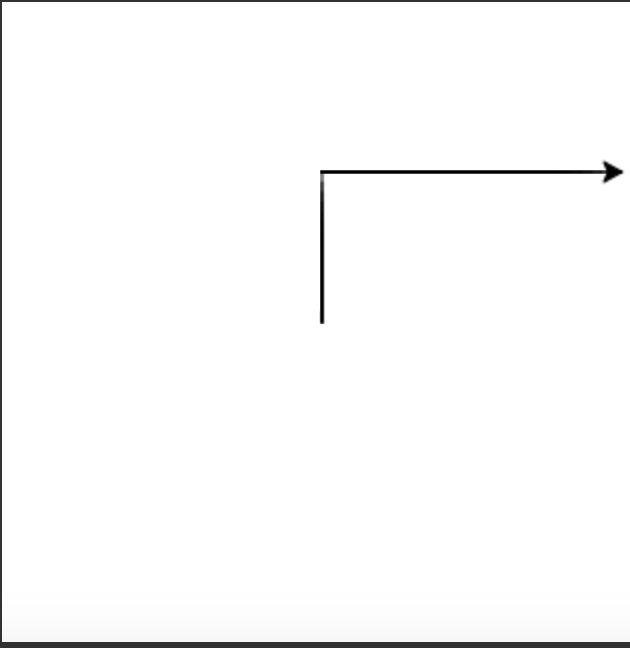• This will draw the shorter line to the north and then the longer one to the east.

6-10-6: What will the following code draw?

```def shape(turtle):
turtle.left(60)
turtle.forward(100)
turtle.right(120)
turtle.forward(100)
turtle.right(120)
turtle.forward(100)
turtle.right(120)

from turtle import *
space = Screen()
luis = Turtle()
shape(luis)
luis.forward(200)
shape(luis)
```
• Two squares connected with a straight line
• This would be true if the right turns were 90 and there were four forwards
• Two triangles connected with a straight line
• This procedure will draw a triangle and it is called twice so it draws two triangles
• Two rectangles connected with a straight line
• This would be true if the right turns were 90 and there were four forwards with two different forward amounts
• Nothing
• This would be true if we only defined the procedure and didn't execute it.